## Example Questions

### Example Question #3 : How To Find The Part From The Whole With Percentage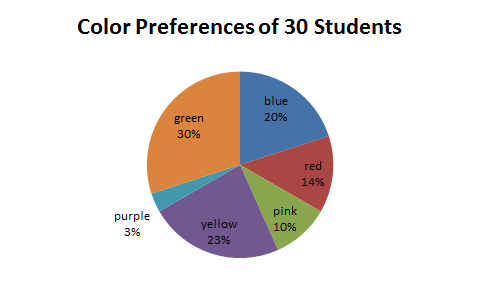The preceding graph depicts students' favorite colors. If there are 30 students in the class, how many prefer blue?

Possible Answers:

7

8

9

6

Correct answer:

6

Explanation:

20% of the students prefer blue. We can calculate the number of students that prefer blue by multiplying the percentage by the total number of students.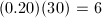### Example Question #4 : How To Find The Part From The Whole With Percentage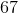is what part of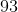?

Round your answer to the nearest percentage.

Possible Answers:Correct answer:Explanation:

To find how much percentis of, simply dividebyand multiply that answer by 100.

Thus,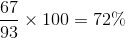### Example Question #4 : How To Find The Part From The Whole With Percentage

The Smith family consumed 35 pounds of table salt last year. As part of a healthier diet, they would like to cut their table salt consumption by 40% this year. In order to reach this goal, what is the maximum amount of salt, in pounds, that the Smith family can consume this year?

Possible Answers:

21

35

42

49

39

Correct answer:

21

Explanation:

We need to first find 40 percent of the amount of table salt that the Smith family consumed in the previous year. In order to calculate this, we need to convert 40 percent into a decimal by moving the decimal place two spaces to the left and multiplying it by last year's salt consumption.Now, we need to subtract this amount from last year's salt consumption in order to calculate this years reduced consumption for the Smith family.### Example Question #1 : Whole And Part

The average cow produces 8 gallons of milk per day. Scientists have found a vitamin supplement that can safely increase a cow’s milk production by 20%. If a dairy farm consisting of 100 cows uses the supplement, how many gallons of milk will the dairy farm now produce? (Assume each cow produces the average amount of milk per day).

Possible Answers:

960 gallons

1000 gallons

160 gallons

800 gallons

Correct answer:

960 gallons

Explanation:

First, determine the amount of milk cows will produce with the supplement: 8 + (.2 x 8)= 9.6 gallons per day. Next, determine the amount of milk 100 cows will produce when receiving the supplement: 100 x 9.6 = 960 gallons.

### Example Question #1 : How To Find The Whole From The Part With Percentage

Florida Clementines Corp sells only the best clementines. The inspector rejected 50% of January’s harvest. Unfortunately, 20% of the inspected clementines fell off the delivery truck. If the truck delivers 200 clementines, how many were harvested in January?

Possible Answers:

350

285

500

80

Correct answer:

500

Explanation:

We first obtain how many clementines were loaded on the delivery truck from 200 delivered clementines. Then we obtain how many clementines were inspected. 200 = (1-0.2)x gives us 250 loaded onto the delivery truck. 250 = (1-0.5)x gives us 500 harvested clementines.

### Example Question #1 : How To Find The Whole From The Part With Percentage

Ed eats 3 cookies every day. If he increases the total amount of cookies he has to begin with by 50%, it would take 21 days to finish all of his cookies. How many cookies does he have to begin with?

Possible Answers:

12 cookies

42 cookies

21 cookies

63 cookies

Correct answer:

42 cookies

Explanation:

We let T be the total amount of cookies and D be the number of days it takes to eat all of the cookies before increasing by 50%, and A be the number of days after increasing by 50%. With the information given, we can write the expressions, T=3D and 1.5T=3A. For the second equation, we know A=21. We plug in and get T=42.

### Example Question #1 : How To Find The Whole From The Part With Percentage

There are 8 blue balls in a bag. 40% of all the balls in the bag are blue. How many total balls are in the bag?

Possible Answers:

20

8

4

12

2

Correct answer:

20

Explanation:

You evaluate 8/0.4 = 20.

### Example Question #1 : How To Find The Whole From The Part With Percentage

95 is 20% of what number?

Possible Answers:

500

475

400

550

Correct answer:

475

Explanation:

multiply the part times 100 then divide by the percent.

(95 x 100) / 20 = 475

### Example Question #1 : How To Find The Whole From The Part With Percentage

If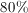of a number is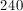, what is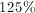of the number?

Possible Answers: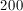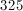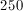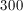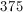Correct answer:Explanation:

Sinceof the number is, the number is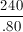which equals. Then calculateof: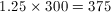### Example Question #1 : How To Find The Whole From The Part With Percentage

Gwen has a collection of 1,000 books.  The average thickness of each book is 1.25” thick.  She wants to buy enough bookshelves that all of her books will fit on it.

What is the shortest length of bookshelf she should buy, in whole feet, to fit all her books?

Possible Answers:

125 feet

105 feet

110 feet

115 feet

120 feet

Correct answer:

105 feet

Explanation:

Length of books = 1,000 books x 1.25” per book = 1,250”

Translate to feet

1” = 12’

1250” / 12 = 104.16 feet

Round up to 105 feet to be able to fit all the books.

### All ACT Math Resources